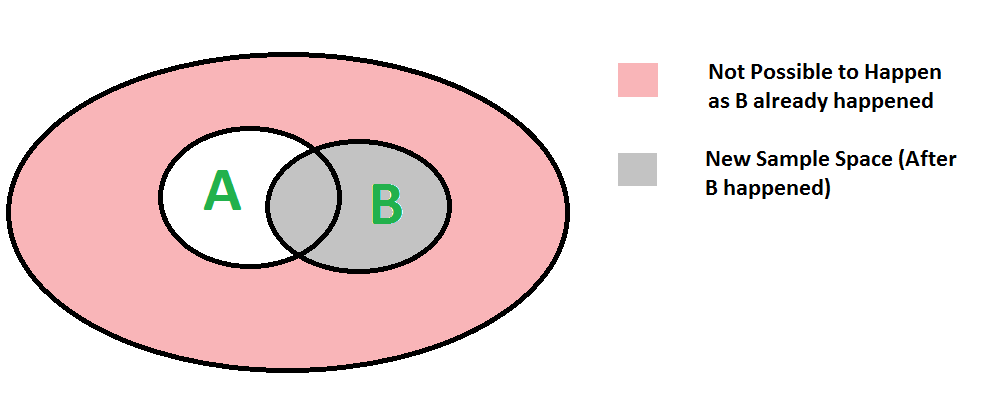# Randomized Algorithms | Set 0 (Mathematical Background)

Conditional Probability Conditional probability P(A | B) indicates the probability of even ‘A’ happening given that the even B happened.We can easily understand above formula using below diagram. Since B has already happened, the sample space reduces to B. So the probability of A happening becomes P(A ? B) divided by P(B)Below is Bayes’s formula for conditional probability.The formula provides relationship between P(A|B) and P(B|A). It is mainly derived from conditional probability formula discussed in the previous post

Consider the below formulas for conditional probabilities P(A|B) and P(B|A)Since P(B ? A) = P(A ? B), we can replace P(A ? B) in first formula with P(B|A)P(A)
After replacing, we get the given formula. Refer this for examples of Bayes’s formula.

Random Variables:
A random variable is actually a function that maps outcome of a random event (like coin toss) to a real value.

Example :

Coin tossing game :
A player pays 50 bucks if result of coin

The person gets 50 bucks if the result is
Tail.

A random variable profit for person can
be defined as below :

-50 if Tail

Generally gambling games are not fair for players,
the organizer takes a share of profit for all
arrangements. So expected profit is negative for
a player in gambling and positive for the organizer.
That is how organizers make money.

Expected Value of Random Variable :
Expected value of a random variable R can be defined as following

    E[R] = r1*p1 + r2*p2 + ... rk*pk

ri ==> Value of R with probability pi

Expected value is basically sum of product of following two terms (for all possible events)
a) Probability of an event.
b) Value of R at that even

Example 1:
In above example of coin toss,
Expected value of profit = 50 * (1/2) +
(-50) * (1/2)
= 0

Example 2:
Expected value of six faced dice throw is
= 1*(1/6) + 2*(1/6) + .... + 6*(1/6)
= 3.5

Linearity of Expectation:
Let R1 and R2 be two discrete random variables on some probability space, then

     E[R1 + R2] = E[R1] + E[R2]

For example, expected value of sum for 3 dice throws is = 3 * 7/2 = 7

Refer this for more detailed explanation and examples.

Expected Number of Trials until Success
If probability of success is p in every trial, then expected number of trials until success is 1/p. For example, consider 6 faced fair dice is thrown until a ‘5’ is seen as result of dice throw. The expected number of throws before seeing a 5 is 6. Note that 1/6 is probability of getting a 5 in every trial. So number of trials is 1/(1/6) = 6.
As another example, consider a QuickSort version that keeps on looking for pivots until one of the middle n/2 elements is picked. The expected time number of trials for finding middle pivot would be 2 as probability of picking one of the middle n/2 elements is 1/2. This example is discussed in more detail in Set 1
Refer this for more detailed explanation and examples.

More on Randomized Algorithms: